Belts

When a belt slides on a surface, the friction between the belt and the surface makes the tension in the belt change. If the belt is sliding on a cylindrical surface, the tension will depend on the contact angle and the coefficient of friction between the belt and the cylinder.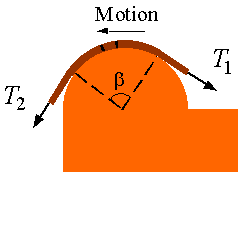To show this we can draw the free body diagram of a segment of the belt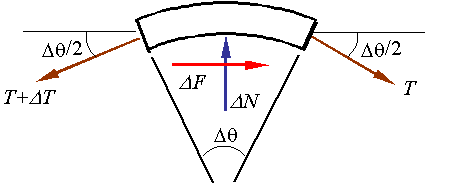The equilibrium equations for this segment are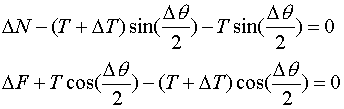Substitution of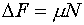, using the small angle approximations, and taking a limit yields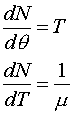Combining the two equations to eliminate N yields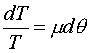Integrating this over the interval of contact yields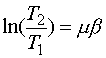The relation between tension on one side of the contact and on the other side can be then calculated from the equation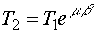where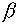is the contact angle in radians and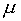is the coefficient of friction.